# Basic Trajectory Prediction in Unity

Over the weekend, Austin Mackrell and I wanted to try out implementing the prediction and visualization of a trajectory in 2-D before it fires. Simply put, we’re firing an object through a gravity affected scene with a certain force, and we want to predict and show the path it would take before we fire it. We saw a ton of articles about it and have seen a number of games implement it in some way. There seemed to be two popular ways to do it, each having its own strengths and weaknesses.

• Option One is to manually calculate how each of the Physics variables, like Force, Mass, Velocity, and Gravity would affect the object. With all the proper information and formulas, one can accurately forecast this.
• Option Two is to create a parallel scene in which physics are drastically accelerated. Every time you show intent to launch the object in your main scene, you’re essentially launching the object in an duplicated scene, which is completing the action at an almost instantaneous speed. Main Scene passes launch information into Duplicate Scene, and Duplicate Scene passes the results of that launch back to Main Scene.

I chose Option One because I like how Math can in theory, perfectly calculate things for us in front of our eyes. Austin chose Option Two, and we thought it’d be interesting to illustrate and contrast the two.

It sounded easy.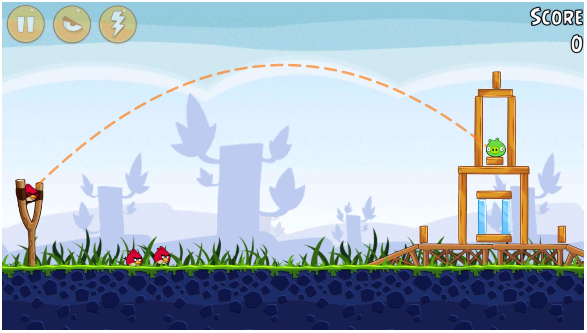Most people will know Trajectory Projection from Angry Birds

It sounded easy until I attempted it. The bad news: there aren’t any mainstream methods of predicting this for us within Unity, we’ll have to build it ourselves. The good news: Many have been here before us. Before video games, before computer simulation and before coding, we’ve had science. Mathematicians and physicists have been at this since long before us. As early as four hundred years ago, Galileo was demonstrating accurate Parabolic Trajectory.

# f(t) = (x0 + x*t, y0 + y*t - g*t²/2)

This is our mother equation.

Looks complicated, I know. Let’s start basic. Take an object moving at a fixed directional speed through a two dimensional space, not being affected by gravity. I fire a bullet upwards at a speed (velocity) of 1x and 2y per second.

The multitude of terms here can get dizzying quick. One helpful thing to note is the synonymity of Vector Velocity and Direction. Our object is traveling 1x and 2y every second, and both of these can be stored in one variable, a Vector2. Therefore, our Vector Velocity is (1ₓ, 2ᵧ) per time unit. Our Direction is also a Vector2 of the same ratio as V.V. The useful distinction is that we’ll normalize the ratio for Direction, which simplifies it into a magnitude of 1. What this boils down to is: Our V.V. is (1ₓ, 2ᵧ), normalized via Unity, returns ~(0.45ₓ, 0.89ᵧ), our Direction. Direction will be particularly useful later when we’re applying Force to our object. Notice they retain the same ratio; Y is twice as much as X. This is a feature in Unity that is inherently used often, even though you may not notice. Ever use transform.up, left, right, down, forward, or back? They’re constantly referenced in Unity, because the information they hold is very useful.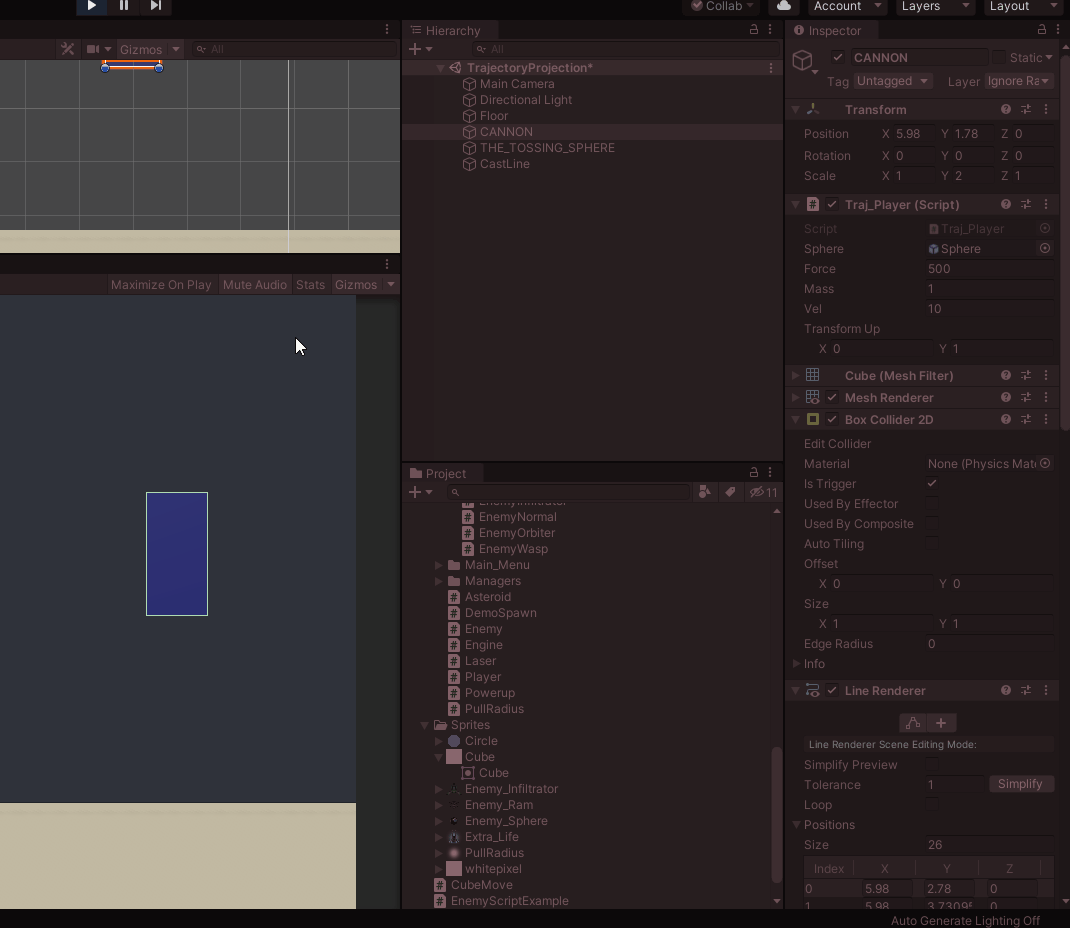Serialization of transform.up for this object. Do it some time and see for yourself. Transform Directions are always returned to us in magnitudes of 1, meaning the maximum value for X and Y are 1, when the other is 0. The same applies for all transform.directions, AKA the red, blue, and green axes of any object.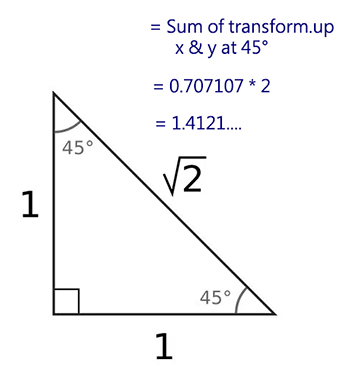TANGENT TIME: Ever wonder why the most optimum angle for launching objects is 45°? Probably not, but I’ll tell you anyway. Notice at the end of the GIF where I set the Rotation to -45°. Transform.Up has X and Y equal at about 0.707 each. Add these together for a sum of ~1.41. No other possible sum of X and Y normalized is greater than 1.41. It’s the best angle for applying force to, because both axes are receiving the highest possible force. If you feel like 1.41 sounds familiar, it’s the square root of two. It’s the length of a hypoteneuse whose Triangle has minor sides equal to 1, with minor angles of, you guessed it, 45°. No coincidence that it’s a significant number here.

Going off of this, we can predict where the object will be by just asking how much Time has passed. I want the position of X after 5 seconds, so I’ll take the Origin of X and add the xVelocity * 5. Do the same to Y.

• x(t) = x0 + x*t
• y(t) = y0 + y*t
• Combine into one Vector2 — f(t) = (x0 + x*t, y0 + y*t)

So at any given point in time, add the Velocity * Time to the Origin.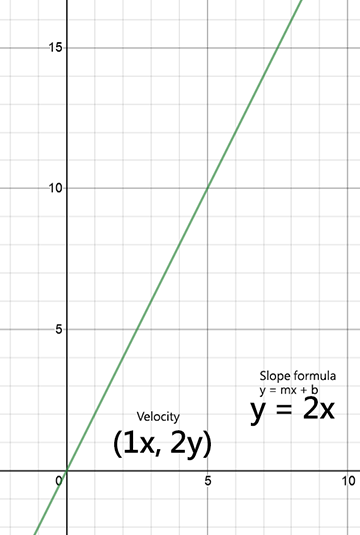The green line represents all positions at a Velocity of 1x, 2y. Converted to slope formula, which is represented in terms of Y, it’s y=2x (b is 0), or Y is traveling twice as fast as X.

Follow the green line. Every second, we’re traveling 1x and 2y. At 2 seconds, we’re at 2x and 4y. At 5, we’re at 5x, 10y.

We can predict every point of this trajectory at any point in time. There are infinite points.

• Origin — Starting point (0,0)
• Velocity — Speed on each axis, i.e. (x, 2y)
• Time unit — Any theoretical unit in time, start in seconds
• Easy so far!!

Now for meat and potatoes. To factor in Gravity, our original equation for the X-Axis will remain constant, we just need to account for Gravity on the Y-Axis. Again, it’s not as bad as it sounds. Remember, we’re standing on the shoulders of scientific giants here. A quick google search for calculating distance affected by gravity yields the necessary equation.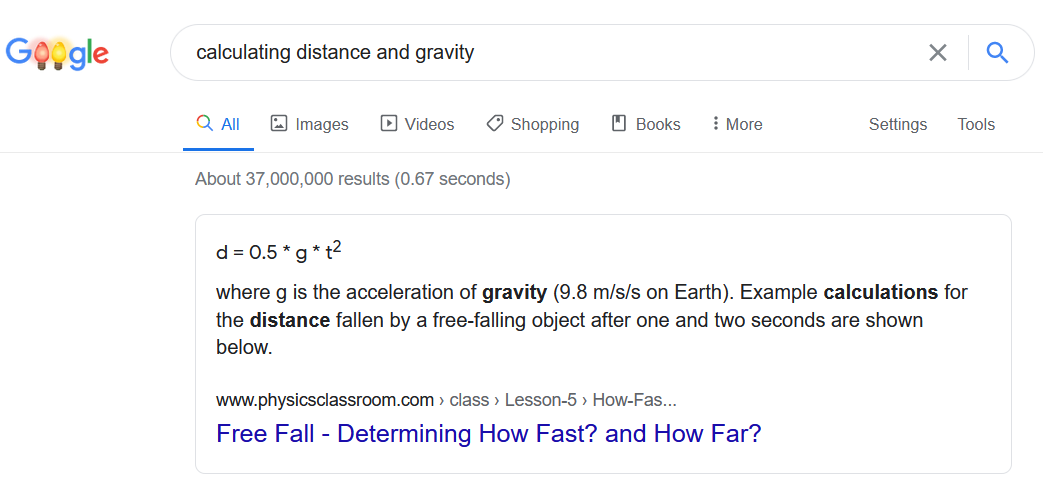Distance = 1/2 * Gravity * Time²

Simply put, add this to the Y-Axis portion of our current distance equation.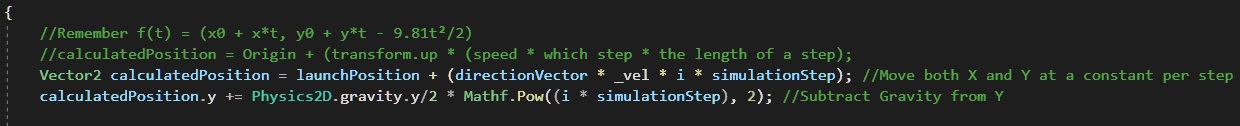See the bones of our equation in there? First line: position on both axes is calculated via a flat rate of velocity * time. Time is represented by (i * simulationStep) which will soon be elaborated on. Second line: Then all we do is subtract Gravity from the Y-Axis (Gravity/2 * time²). Remember Gravity is -9.81 (IRL 9.81m/s²). Debug.Log it in Unity sometime to see for yourself!

All we’re doing is breaking our initial equation down into smaller pieces.

• Position in Time = Origin + (Direction * Speed * Time)
• Y Position = SAME AS ABOVE minus Gravity/2 * time²
• f(t) = (x0 + x*t, y0 + y*t - g*t²/2)

On track so far? One last thing, We’d like to be able to figure out Velocity based upon a possibly constant changing of Direction and Force and Mass. No sweat — shoulders of giants — google what you need.

• Velocity = Acceleration × Time
• Acceleration = Force ÷ Mass
• Thus, Velocity = Force / Mass * Time

Also important to note here: Velocity in this instance gives us the Velocity Modifier by which we will multiply Direction to get our Vector Velocity. While I think Vector Velocity was important to point out, we can disregard it for our intents and purposes and just remember Velocity Modifier is Velocity, a float. Maybe knowing this in advance will help you avoid confusion in the future. Remember, Velocity will be given to us in the units of Time that we choose. Take Unity’s built in Time units.

• Time.deltaTime is the amount of time it took to execute the previous frame, usually some fraction of a second
• Time.fixedDeltaTime is the fixed interval of time in which the Unity Physics system will iterate. Default value: 0.02s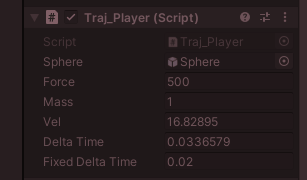SERIALIZE AND DEBUG EVERYTHING FOR BIG KNOWLEDGE

I’m trying to calculate Velocity here via time.deltaTime. Not working, because deltaTime is all over the place, but look at steady old fixedDeltaTime over there. When using Physics in Unity, use fixedDeltaTime.

Bring it all together! Velocity = Force / Mass * Time.fixedDeltaTime

More cleanly shown; Velocity for an object of Mass 1 having a Force of 500 applied to it equals 10.

We’re close to bringing everything together here. We want a trajectory to plot positions at certain intervals in time, and draw a line between them. For performance reasons, we don’t want our simulation logging infinite amount of points, even though there are. We want the smallest amount of points drawn that will still accurately portray a trajectory to our liking. Let’s start relatively small: an interval of 0.1f, or 10 measurements every simulated second. Remember too, our simulation doesn’t know when to stop. Let’s start at 5 simulated seconds.

You’re going to have to decide how you want Force and Direction applied in your implementation. I’ll start with fixed amounts and include code for a more complex iteration as well. Notice all of our relevant variables below:

• Total duration of simulation
• Time interval of each Step
• Maximum potential amount of Steps
• Launch Direction
• Launch Origin
• Velocity = Force / Mass * Time.fixedDeltaTime
• Position (at time) = Origin + Direction * Velocity * Time (i * step)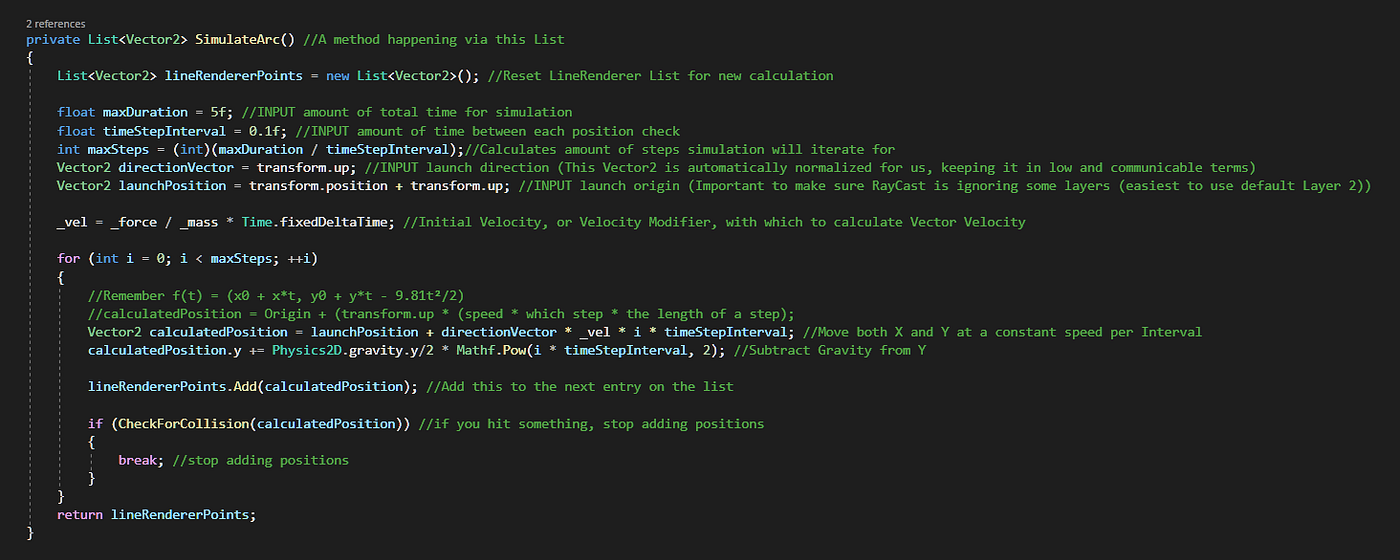Be careful with order of operations. PEMDAS. If in doubt, parenthesize everything to ensure the equation is happening in your intended order.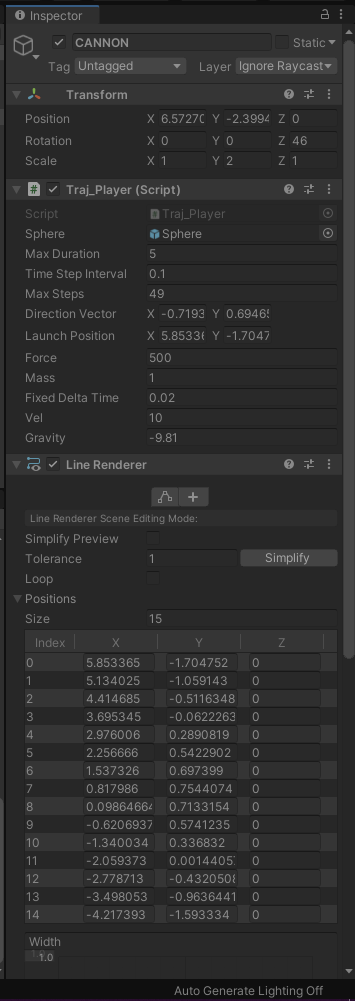Code, serialized variables for reference and visual of the working trajectory! All the necessary tools with which to equate points in time for trajectory visualization.

In its current iteration, our LineRenderer is calculating positions in intervals of 0.1s.

Our current LineRenderer could potentially be recalculating 49 different positions of trajectory every frame! Useful to include in code is a Collision measurement each time a new point is measured. If it collides, it stops calculating new points. Ours calculates a new position every tenth of a second until it collides with an object at Point 14, and stops calculating.

I won’t be explaining the LineRenderer code for want of keeping the article simple.

Force and Direction implementation are up to you. Included below are two methods of code. A cannon style trajectory, and a click/drag to fling trajectory.

The world is your oyster.

I’ve tried to break things down in a way that’s most understandable, but this is of course an equation can be done in fewer steps and with different variables which essentially communicate the same information. You may see this explained in different terms. Fret not, remember the gist.

• f(t) = (x0 + x*t, y0 + y*t - 9.81t²/2)
• Velocity = Force / Mass * Time.fixedDeltaTime

This is the most basic implementation of trajectory projection in Unity. Option One, manual calculation, is the more performance optimized method, and potentially the most pure if all factors are carefully (and painstakingly) accounted for. Some things it doesn’t account for currently, which you will notice pile up pretty quickly as far as one central mathematical computation goes, adding to the difficulty:

• Rigidbody components like Gravity Scale & Drag. These can be calculated relatively easily.
• 3-Dimensional Space, although the Z-Axis would act just like the X-Axis, with another simple flat velocity equation. Relatively easy to add
• Objects in motion. Again, possible, but beginning to get more tediously involved in an active understanding of the Physics process
• Bounces — Bounces, and other collider behavior like ground speed (angular drag), are a major shortfall of the manual mathematical implementation of trajectory projection. To calculate bounces would take a considerable amount of dissection of the Unity Physics program and the properties of each potential Rigidbody that gets bounced or bounced off of. This is the major benefit of the Parallel Scene implementation of trajectory projection. You will see this implemented in things like Billiards games.

Option Two, while more performance intensive, can easily cover all the things that make Manual Calculation so daunting and complicated. Explained better in Austin’s article, linked below.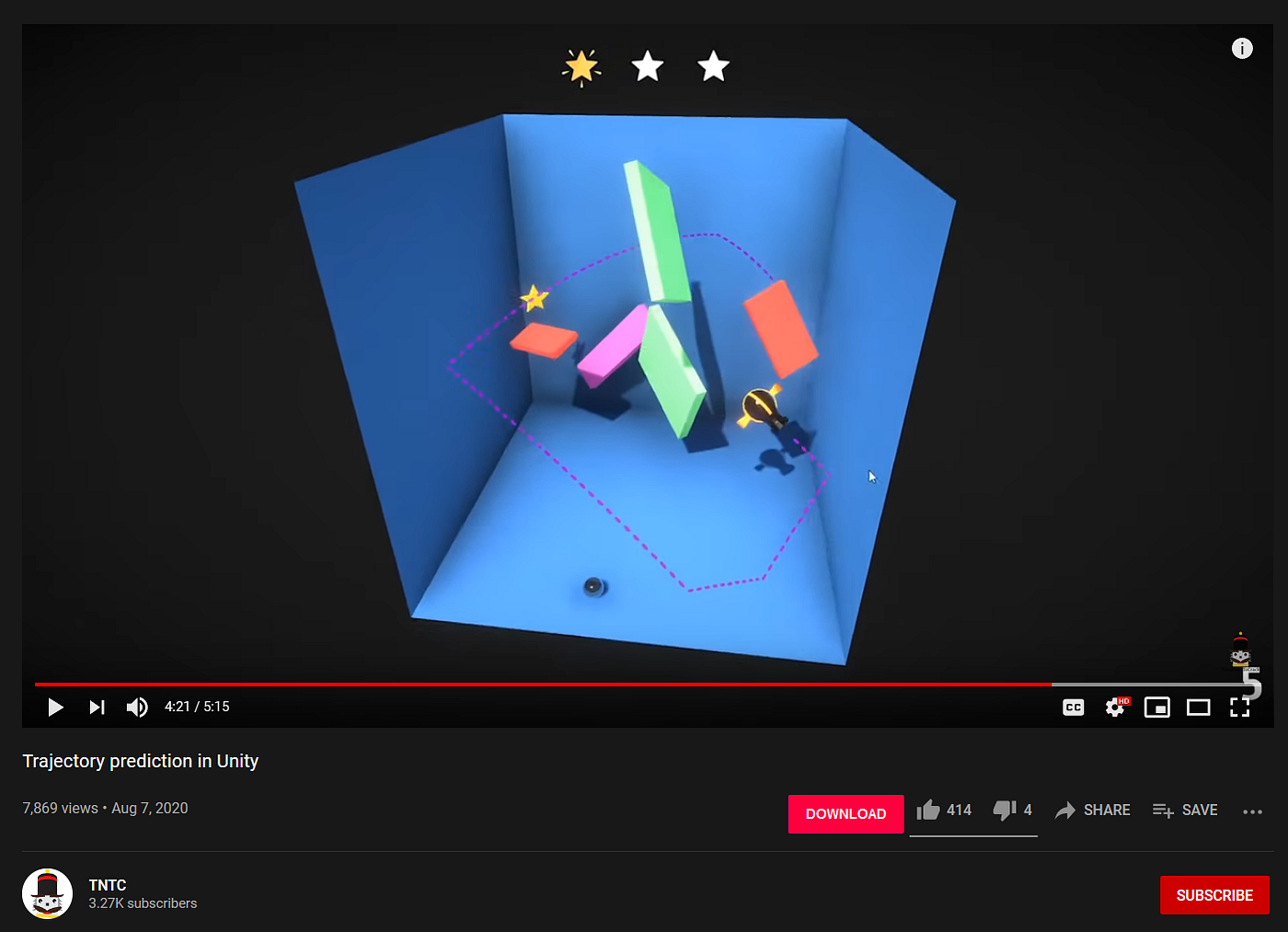Wonderful bouncing demonstration via ToughNutToCrack. With Parallel Scene simulation, you can more easily predict behavior like bouncing in addition to matching other exact Physics components.

To budding developers: Yes, I learned all of this recently from scratch by scouring through different explanations and thoroughly debugging through trial and error. The overlying lesson here is: you can do the same with other features in software development, or generally anything new in life. Remember the power of Google and the brave soldiers who came before you with similar questions. Ability to research and analyze is your most important skill as a developer. The great thing is, these skills are not talent-based, rather manifested through simple, diligent, patient observation coupled with good old fashioned mimicry. Fake it ’til you make it people!

My aim in all of this is to show the method, not gift it. There are of course necessary components to assign within Unity, but if you’ve made it this far, the worst is behind you.

Questions welcome. Will edit article as needed.

--

--